/

### Function Description

Returns a two element array containing as its first termand its second term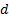which is the canonically simplified form of the input fraction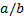where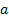and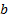are both integral and the input is in the form of two separate integers,andrespectively.

The canonically simplified form of such a fraction is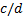whereis positive and the greatest common divisor of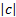andis 1.

Contents | Prev | Next